## Windows Phone开发（5）：室内装修

junwong 发布于 2012/04/18 14:50

为什么80%的码农都做不了架构师？->>>1、启动VS，新建一个WP应用程序，不用我多说了，都会了。

2、删除页面中的根Grid，整个删除。如下图所示。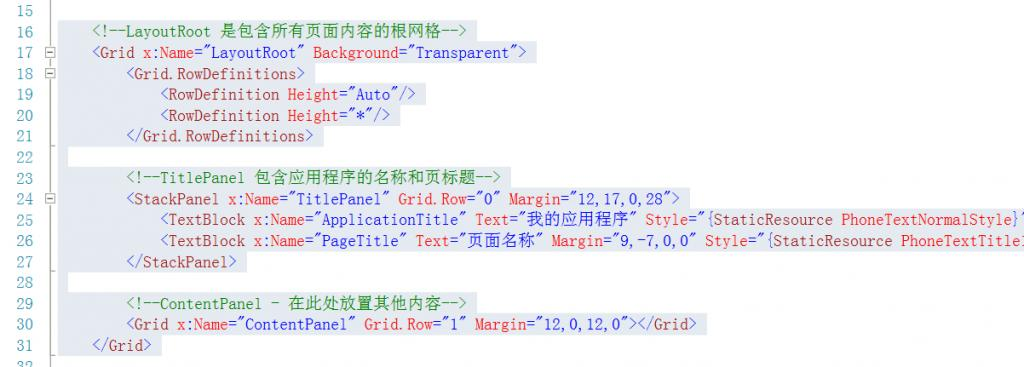3、然后，替换为以下XAML代码。

```    <Grid x:Name="Root">
<Grid.ColumnDefinitions>
<ColumnDefinition Width="*"/>
<ColumnDefinition Width="*"/>
</Grid.ColumnDefinitions>
<Grid.RowDefinitions>
<RowDefinition Height="*"/>
<RowDefinition Height="*"/>
</Grid.RowDefinitions>
</Grid>```

（1）可以用数值，double类型，如120.667，这个数字与屏幕分辨率无关，运行运行库会自行调整；

（2）*：这个星号是什么意思呢？就像上面的例子，我都用了*，这么说是说不清楚的，我举个例子吧。

```    <Grid x:Name="Root" ShowGridLines="True">
<Grid.RowDefinitions>
<RowDefinition Height="2*"/>
<RowDefinition Height="*"/>
<RowDefinition Height="3*"/>
</Grid.RowDefinitions>
</Grid>```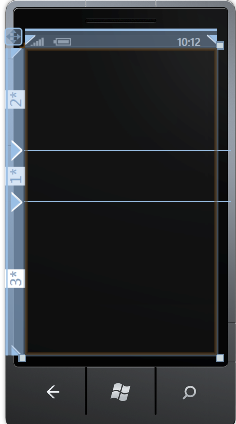```    <Grid x:Name="Root" ShowGridLines="True">
<Grid.RowDefinitions>
<RowDefinition Height="3*"/>
<RowDefinition Height="7*"/>
</Grid.RowDefinitions>
</Grid>```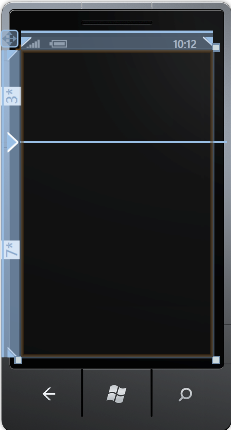```    <Grid x:Name="Root" ShowGridLines="True">
<Grid.RowDefinitions>
<RowDefinition Height="86"/>
<RowDefinition Height="5*"/>
<RowDefinition Height="3*"/>
</Grid.RowDefinitions>
</Grid>```

（3）Auto，不用多解释，从单词的含义就知道了，就是根内容自动调整。

```    <Grid x:Name="Root" ShowGridLines="True">
<Grid.RowDefinitions>
<RowDefinition Height="*"/>
<RowDefinition Height="*"/>
</Grid.RowDefinitions>
<Grid.ColumnDefinitions>
<ColumnDefinition Width="*"/>
<ColumnDefinition Width="*"/>
</Grid.ColumnDefinitions>
<!-- 内容 -->
<TextBlock Grid.Column="0" Grid.Row="0" Text="第1行第1列" FontSize="35"/>
<Rectangle Fill="Yellow" Grid.Column="1" Grid.Row="0" Margin="68"/>
<Button Grid.Column="0" Grid.Row="1" Content="第2行第1列" FontSize="32"/>
<Ellipse Fill="Blue" Height="95" Width="180" Grid.Column="1" Grid.Row="1"/>
</Grid>```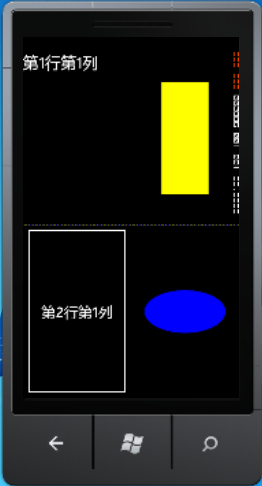```    <StackPanel Orientation="Horizontal" Height="100">
<Button Content="按钮1"/>
<Button Content="按钮2"/>
<Button Content="按钮3"/>
</StackPanel>```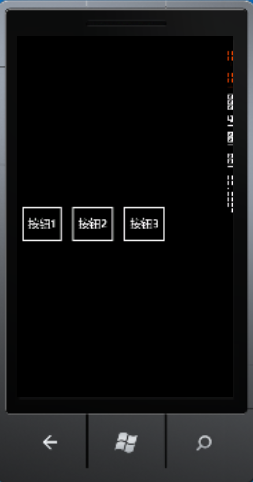```    <StackPanel Orientation="Vertical" Width="300">
<TextBlock Text="文本一" FontSize="80"/>
<TextBlock Text="文本二" FontSize="80"/>
<TextBlock Text="文本三" FontSize="80"/>
</StackPanel>```

（图6）运行效果如下：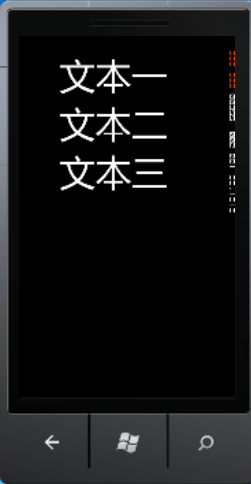```    <Canvas>
<Rectangle Fill="Orange" Canvas.Left="37" Canvas.Top="116" Height="165" Width="220" />
<Path Data="M0,0 L0,8 L12,8 Z" Fill="Silver"
Canvas.Left="127"
Canvas.Top="204"
Width="260"
Height="235" Stretch="Fill"/>
</Canvas>```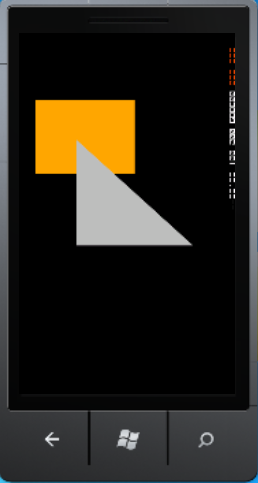```    <Canvas>
<Rectangle Fill="Orange" Canvas.Left="37" Canvas.Top="116"
Height="165" Width="220"
Canvas.ZIndex="1"/>
<Path Data="M0,0 L0,8 L12,8 Z" Fill="Silver"
Canvas.Left="127"
Canvas.Top="204"
Width="260"
Height="235" Stretch="Fill"/>
</Canvas>```